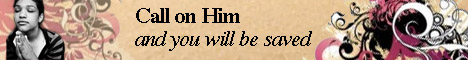Note:  Do not rely on this information. It is very old.

# Denary Scale

Denary Scale is the scale of notation in general use for the expression of number. The base of the system is the number 10, the position of the digits indicating the power of 10 by which each is multiplied. Thus the expression 3574 signifies, in the denary scale, the number 3000 + 500 + 70 + 4, the 3 being multiplied by the cube of 10, the 5 by the square of 10, and so on. The system of decimal fractions is thus only an extension of the denary scale to represent fractional numbers.